# NCERT solutions for Science Exemplar Class 6 chapter 10 - Motion and Measurement of Distances [Latest edition]

#### Chapters## Chapter 10: Motion and Measurement of Distances

Multiple Choice Questions [Pages 56 - 60]

### NCERT solutions for Science Exemplar Class 6 Chapter 10 Motion and Measurement of Distances Multiple Choice Questions [Pages 56 - 60]

Multiple Choice Questions | Q 1. | Page 56

The distance between Delhi and Mumbai is usually expressed in units of

• decametre

• metre

• centimetre

• kilometre

Multiple Choice Questions | Q 2. | Page 56

Which of the following does not express a time interval?

• A day

• A second

• A school period

• Time of the first bell in the school

Multiple Choice Questions | Q 3. | Page 56

Fig. shows a measuring scale which is usually supplied with a geometry box. Which of the following distance cannot be measured with this scale by using it only once?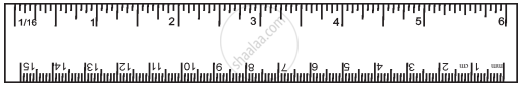• 0.1 m

• 0.15 m

• 0.2 m

• 0.05 m

Multiple Choice Questions | Q 4. | Page 57

A piece of ribbon folded five times is placed along a 30 cm long measuring scale as shown in Fig.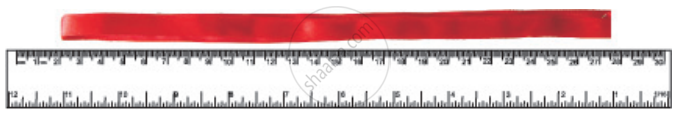The length of the ribbon is between

• 1.15m - 1.25m

• 1.25m - 1.35m

• 1.50m - 1.60m

• 1.60m - 1.70m

Multiple Choice Questions | Q 5. | Page 57

Paheli moves on a straight road from point A to point C. She takes 20 minutes to cover a certain distance AB and 30 minutes to cover the rest of distance BC. She then turns back and takes 30 minutes to cover the distance CB and 20 minutes to cover the rest of the distance to her starting point. She makes 5 rounds on the road the same way. Paheli concludes that her motion is

• only rectilinear motion.

• only periodic motion.

• rectilinear and periodic both.

• neither rectilinear nor periodic.

Multiple Choice Questions | Q 6. | Page 58

Bholu and Golu are playing in the ground. They start running from the same point A in the ground and reach point B at the same time by following the paths marked 1 and 2 respectively as shown in Fig. Which of the following is/are true for the given situation.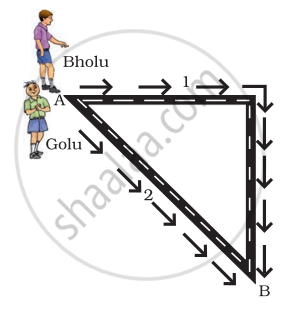As compared to Golu, Bholu covers a

• longer distance but with a lower speed.

• longer distance with a higher speed.

• shorter distance with a lower speed.

• shorter distance with a higher speed.

Multiple Choice Questions | Q 7. | Page 58

Four pieces of wooden sticks A, B, C, and D are placed along the length of 30 cm long scale as shown in Fig. Which one of them is 3.4 cm in length?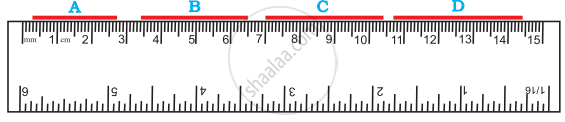• A

• B

• C

• D

Multiple Choice Questions | Q 8. | Page 58

Which of the following figure shows the correct placement of a block along a scale for measuring its length?

•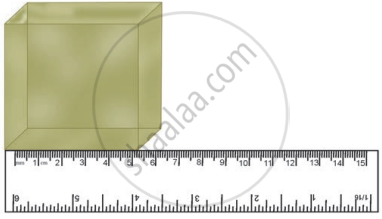•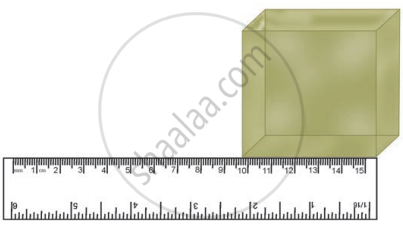•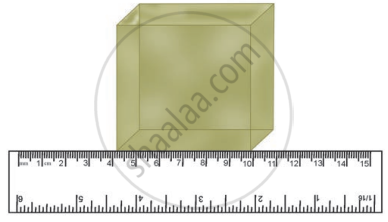•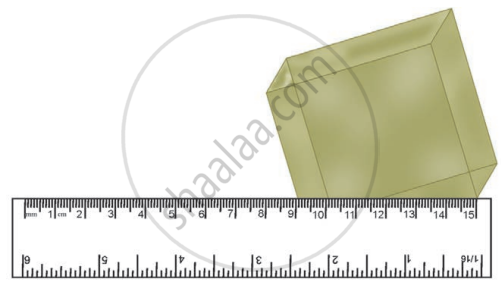Multiple Choice Questions | Q 9. | Page 60

You are provided three scales A, B, and C as shown in Fig. to measure a length of 10 cm.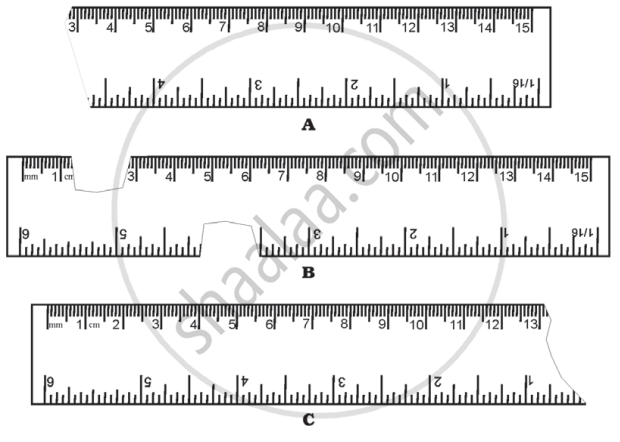For the correct measurement of the length you will use the scale

• A only

• B only

• C only

• Any of the three scales

Very Short Answer Questions [Page 61]

### NCERT solutions for Science Exemplar Class 6 Chapter 10 Motion and Measurement of Distances Very Short Answer Questions [Page 61]

Very Short Answer Questions | Q 10. (i) | Page 61

Correct the following.

The motion of a swing is an example of rectilinear motion.

Very Short Answer Questions | Q 10. (ii) | Page 61

Correct the following.

1m = 1000 cm

#### Fill in the blanks

Very Short Answer Questions | Q 11. (i) | Page 61

Motion of an object or a part of it around a fixed point is known as ______ motion.

Very Short Answer Questions | Q 11. (ii) | Page 61

A body repeating its motion after a certain interval of time is in ______ motion.

Very Short Answer Questions | Q 11. (iii) | Page 61

In rectilinear motion, the object moves ______ a ______ line.

Very Short Answer Questions | Q 11. (iv) | Page 61

SI unit of length is ______

Very Short Answer Questions | Q 12. (i) | Page 61

Write one example of the following type of motion.

Rectilinear

Very Short Answer Questions | Q 12. (ii) | Page 61

Write one example of the following type of motion.

Circular

Very Short Answer Questions | Q 12. (iii) | Page 61

Write one example of the following type of motion.

Periodic

Very Short Answer Questions | Q 12. (iv) | Page 61

Write one example of the following type of motion.

Circular and periodic

### NCERT solutions for Science Exemplar Class 6 Chapter 10 Motion and Measurement of Distances Short Answer Questions [Page 62]

Short Answer Questions | Q 13. | Page 62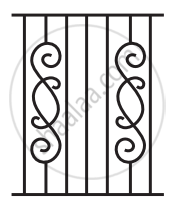The photograph given in figure shows a section of a grille made up of straight and curved iron bars. How would you measure the length of the bars of this section, so that the payment could be made to the contractor?

Short Answer Questions | Q 14. | Page 62

Identify the different types of motion in the following word diagram given.

 Y O U N G C C N T E R L E V E L P I E E A R A L L O T O P P E A I N O T E P A D N E C K O W O N E W I Y Z S E I E V O R L O A D W P T R G N I C E D R I L A Z H T O N G U E N A T X C R D E P T H G R O E Y C I R C U L A R R T L C C O P P E R T
Short Answer Questions | Q 15. | Page 62

Four children measure the length of a table which was about 2 m. Each of them used different ways to measure it.

1. Sam measured it with a half metre long thread.
2. with a 15 cm scale from her geometry box.
3. Reena measured it using her hand span.
4. Salim measured it using a 5 m long measuring tape.

Which one of them would get the most accurate length? Give reason for your answer.

Short Answer Questions | Q 16. | Page 62

Match the events related to motion in column I with the types of motions given in column II.

 Column I Column II (a) A moving wheel of a sewing machine (i) Circular motion (b) Movement of tip of the minute hand of a clock in one hour (ii) Rotational motion (c) A moving swing (iii) Periodic motion

### NCERT solutions for Science Exemplar Class 6 Chapter 10 Motion and Measurement of Distances Long Answer Questions [Page 63]

Long Answer Questions | Q 17. | Page 63

While travelling in a train, it appears that the trees near the track are moving whereas co-passengers appear to be stationary. Explain the reason.

Long Answer Questions | Q 18. | Page 63

How are the motions of a wheel of a moving bicycle and a mark on the blade of a moving electric fan different? Explain.

Long Answer Questions | Q 19. | Page 63

Three students measured the length of a corridor and reported their measurements. The values of their measurements were different.

What could be the reason for difference in their measurements? (Mention any three)

Long Answer Questions | Q 20. | Page 63

Boojho was riding in his bicycle along a straight road. He classified the motion of various parts of the bicycle as

1. rectilinear motion,
2. circular motion and
3. both rectilinear as well as circular motion.

Can you list one part of the bicycle for each type of motion? Support your answer with reason.

## Chapter 10: Motion and Measurement of Distances## NCERT solutions for Science Exemplar Class 6 chapter 10 - Motion and Measurement of Distances

NCERT solutions for Science Exemplar Class 6 chapter 10 (Motion and Measurement of Distances) include all questions with solution and detail explanation. This will clear students doubts about any question and improve application skills while preparing for board exams. The detailed, step-by-step solutions will help you understand the concepts better and clear your confusions, if any. Shaalaa.com has the CBSE Science Exemplar Class 6 solutions in a manner that help students grasp basic concepts better and faster.

Further, we at Shaalaa.com provide such solutions so that students can prepare for written exams. NCERT textbook solutions can be a core help for self-study and acts as a perfect self-help guidance for students.

Concepts covered in Science Exemplar Class 6 chapter 10 Motion and Measurement of Distances are Story of Transport, Measurements, Unit and Its Types, Unit Systems, International System of Units (SI System), Devices for Measuring Length, Measuring the Length of a Curved Line, Motion and Rest, Types of Motion, Multiple Motion.

Using NCERT Class 6 solutions Motion and Measurement of Distances exercise by students are an easy way to prepare for the exams, as they involve solutions arranged chapter-wise also page wise. The questions involved in NCERT Solutions are important questions that can be asked in the final exam. Maximum students of CBSE Class 6 prefer NCERT Textbook Solutions to score more in exam.

Get the free view of chapter 10 Motion and Measurement of Distances Class 6 extra questions for Science Exemplar Class 6 and can use Shaalaa.com to keep it handy for your exam preparation